# IBPS Clerk Question

7. If ‘A’ is coded as 1, ‘B’ as 3, ‘C’ as 5 and so on, which of the following is the numerical value of the word ‘FAZED’? 1) 81 2) 79 3) 77 4) 80 5) None of these ibps clerk question 8. Which of the following pairs of words have the same relationship as FAN : HEAT? 1) Water : Drink 2) Light : Night 3) Teach : Student 4) Air : Breathe 5) Food : Hunger 9. Q types faster than R but not as fast as V. T types faster than R. S types faster than V. Who amongst the five types the fastest? 1) V 2) T 3) S 4) Data Inadequate 5) None of these 10. If ‘B × C’ means ‘B is the daughter of C’, ‘B + C’ means ‘B is the husband of C’ and ‘B - C’ means ‘B is the sister of C’, then what does ‘M + N - P × Q’ mean? 1) M is the brother-in-law of Q. 2) M is the uncle of Q. 3) M is the son-in-law of Q. 4) Q is the mother-in-law of M. 5) None of these Directions (Q. 11-15): Study the following arrangement carefully and answer the questions given below: R D A K 5 B I 2 M J E N 9 7 U Z V 1 W 3 H 4 F Y 8 P 6 T G 11. How many such numbers are there in the above arrangement, each of which is immediately preceded by a consonant and immediately followed by a vowel? 1) None 2) One 3) Two 4) Three 5) More than three 12. Which of the following is the eighth to the left of the seventeenth from the left end? 1) M 2) J 3) 8 4) 5 5) None of these 13. Four of the following five are alike in a certain way based on their positions in the above arrangement and so form a group. Which is the one that does not belong to that group? 1) E 9 J 2) Z 1 U 3) H W 4 4) Y 4 8 5) B 2 K 14. Which of the following is the sixth to the right of the nineteenth from the right end? 1) 5 2) Z 3) V 4) 1 5) None of these
Practice Exercise - 41 [ Bank Reasoning ]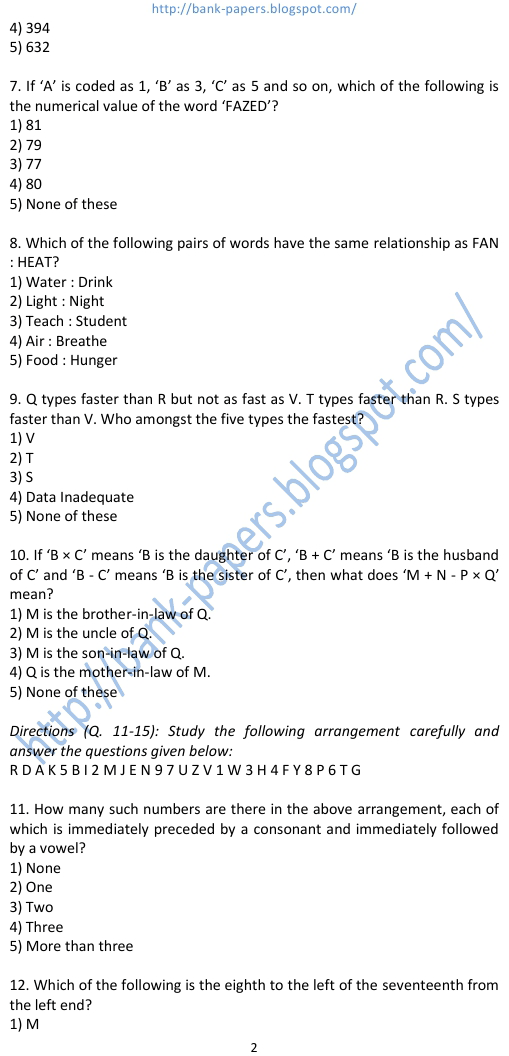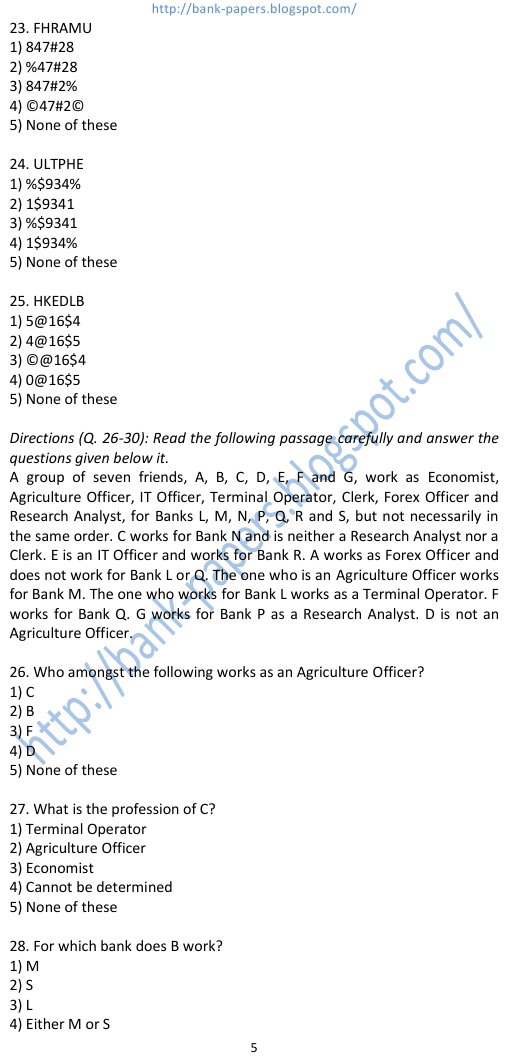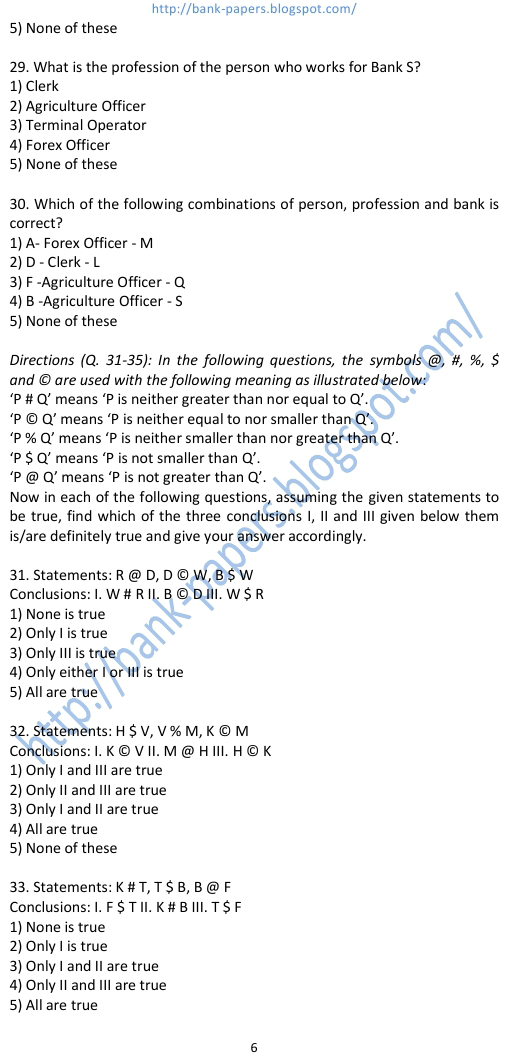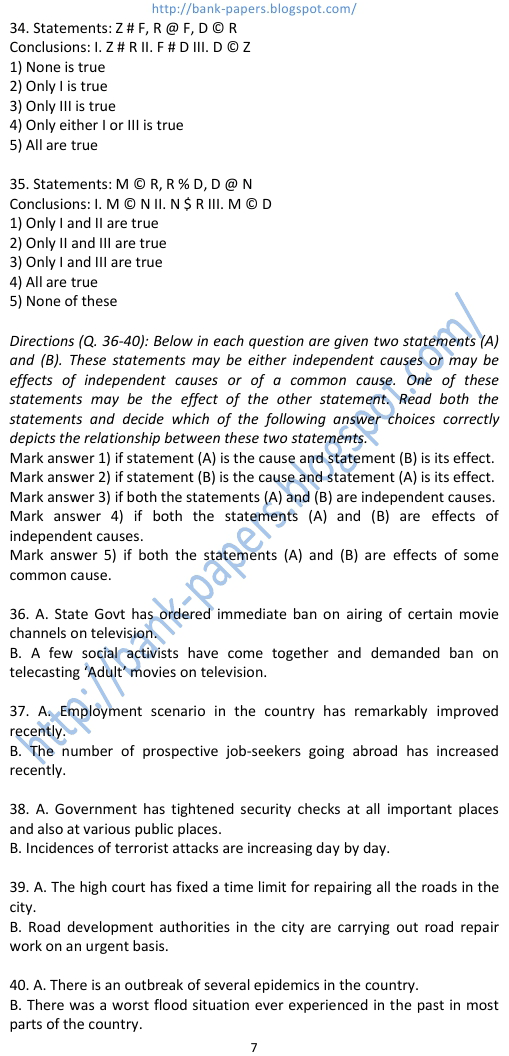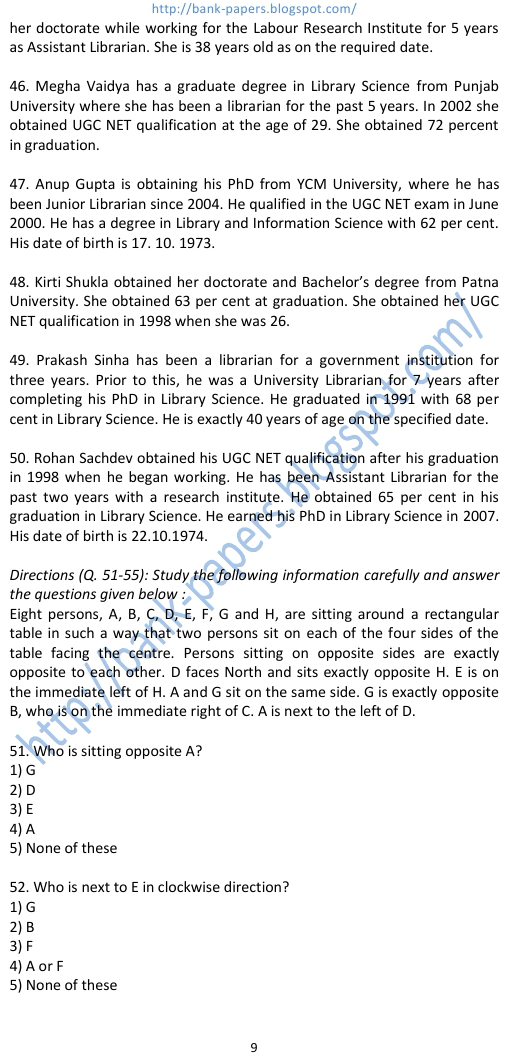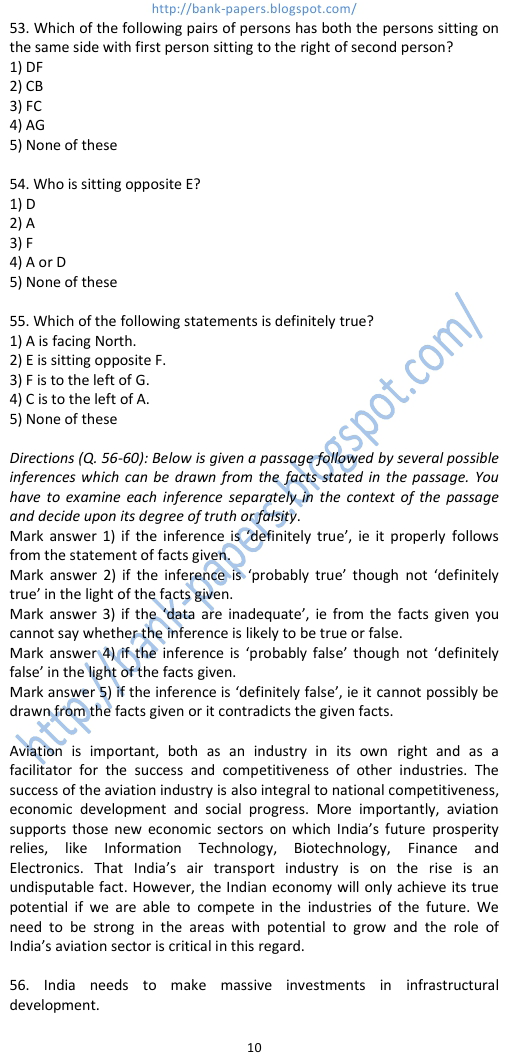## ibps clerk question

### ibps computer question

#### ibps exam

##### ibps exam questions
###### ibps marketing question
banking question for ibps. ibps clerk question. ibps computer question. ibps exam. ibps exam questions. ibps marketing question. ibps model papers. ibps model question. ibps po question. ibps question bank. ibps question booklet. ibps question paper. ibps question papers. ibps question pattern. ibps questions ibps reasoning question. ibps sample questions.